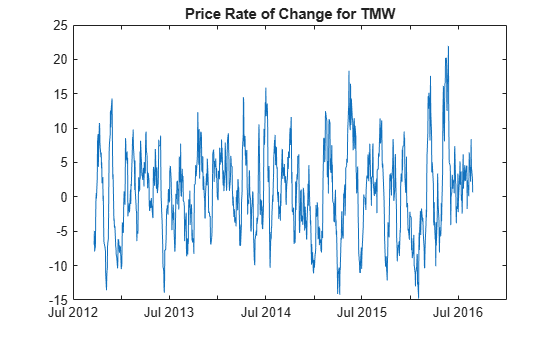# prcroc

Price rate of change

`prcroc` has been partially removed and will no longer accept a `fints` object (`tsobj`) argument. Use a matrix, `timetable`, or `table` instead for financial time series.

Use `fts2timetable` to convert a `fints` object to a `timetable` object.

## Syntax

``PriceChangeRate = prcroc(Data)``
``PriceChangeRate = prcroc(___,Name,Value)``

## Description

example

````PriceChangeRate = prcroc(Data)` calculates the price rate-of-change, `PriceChangeRate`, from the series of closing stock prices. By default, the volume rate-of-change is calculated between the current closing price and the closing price 12 periods ago.```

example

````PriceChangeRate = prcroc(___,Name,Value)` adds optional name-value pair arguments. ```

## Examples

collapse all

Load the file `SimulatedStock.mat`, which provides a timetable (`TMW`) for financial data for TMW stock.

```load SimulatedStock.mat PriceChangeRate = prcroc(TMW); plot(PriceChangeRate.Time,PriceChangeRate.PriceRoc) title('Price Rate of Change for TMW')```## Input Arguments

collapse all

Data for closing prices, specified as a matrix, table, or timetable. For matrix input, `Data` is an `M`-by-`1` matrix of closing prices. Timetables and tables with `M` rows must contain a variable named `'Close'` (case insensitive).

Data Types: `double` | `table` | `timetable`

### Name-Value Pair Arguments

Specify optional comma-separated pairs of `Name,Value` arguments. `Name` is the argument name and `Value` is the corresponding value. `Name` must appear inside quotes. You can specify several name and value pair arguments in any order as `Name1,Value1,...,NameN,ValueN`.

Example: ```PriceChangeRate = prcroc(TMW,'NumPeriods',18)```

Period difference, specified as the comma-separated pair consisting of `'NumPeriods'` and a scalar positive integer.

Data Types: `double`

## Output Arguments

collapse all

Closing price rate-of-change, returned with the same number of rows (`M`) and the same type (matrix, table, or timetable) as the input `Data`.

 Achelis, S. B. Technical Analysis from A to Z. Second Edition. McGraw-Hill, 1995, pp. 243–245.